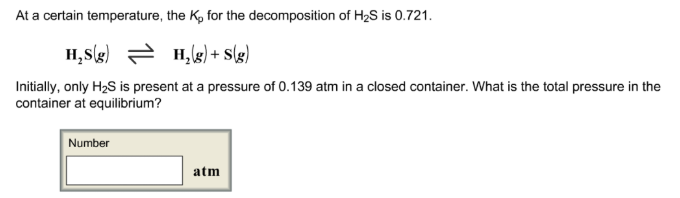# Problem: At a certain temperature, the Kp for the decomposition of H2S is 0.721. H2S(g) ⇌ H2(g) + S(g)Initially, only H2S is present at a pressure of 0.139 atm in a closed container. What is the total pressure in the container at equilibrium?

###### FREE Expert Solution
100% (476 ratings)###### Problem Details

At a certain temperature, the Kp for the decomposition of H2S is 0.721. H2S(g) ⇌ H2(g) + S(g)Initially, only H2S is present at a pressure of 0.139 atm in a closed container. What is the total pressure in the container at equilibrium?